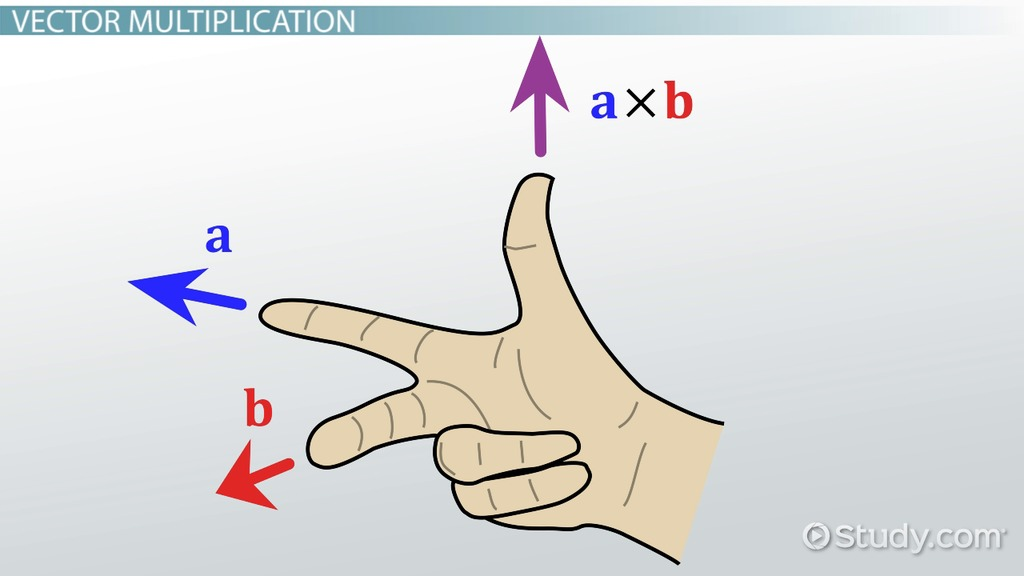# JoyOfVex9

dot and cross product, fake lighting combing normals to a surface

### Day 9

#### Dot product

Switch to a more complex model now, like tommy, turn off textures so we're not distracted by that.

```@Cd = @N.y;
```

That looks like he's lit from above. If you use @N.x or @N.z, he looks lit from the right or front. Can negate to make him look like he's lit from underneath/left/behind.

```@Cd = -@N.y;
```

So thats fine, but what if we want him lit from an arbitrary direction? This is where a dot product comes in handy. It compares the directions of vectors, if they are pointing the same way (ie, parallel), it returns -1. If they're perpendicular, 0, if facing in exact opposite directions, 1, and angles in between those extreme positions will smoothly vary between 1, 0, -1.

Eg, compare the normals to a vector pointing along the y axis {0,1,0}:

```@Cd = dot(@N, {0,1,0});
```

Replace that hard coded vector with a chv slider, now we can light from any angle:

```@Cd = dot(@N, chv('angle'));
```

Adjusting angles with vectors can be a little tricky if you're not used to it, think of it as 'how much x, how much y, how much z', so for a light to come from the left, slightly up, and to the front, would be a value like {1, 0.1, 1}.

An alternative way to think about this is if you had a point in space. You move that where you want, draw a line from the origin to the point, thats your light vector. You can do exactly this; make a single point with an add sop, feed it to the second input, retrieve its @P, and treat it as a vector to do the dot product test:

```vector pos = point(1,'P',0);
@Cd = dot(@N, pos);
```

The point function here goes to input 1, and retrieves the @P attribute of point 0. Note that there's no @ prefix, just to keep you on your toes. :)

This works, but the light gets brighter as the point gets further away. As I mentioned a while back, a lot of functions expect the input vectors to be normalised, this is one of those cases.

```vector pos = point(1,'P',0);
pos = normalize(pos);
@Cd = dot(@N, pos);
```
```==== Cross product ====
```

Switch to a sphere now. Turn on 'view point normals' in the viewport. You see a ball with spikes pointing away from it. Check what happens here:

```@N = cross(@N, {0,1,0});
```

It can be a little hard to see against the mesh, append an add sop, turn on 'delete geometry but keep points', to make it easier to see. The normals are now rotating around the surface of the sphere.

The cross product will take 2 vectors, and generate a 3rd perpendicular vector. Easiest to think about with normals at the equator, and doing a vector vulcan hand shape () . Point your thumb up along the y axis, middle finger along a normal at the equator, your index finger now points along the equator. Turn your hand to represent normals at various points around the equator, you can see that it will swirl the normals (or 'combs' the normals as this operation is sometimes called) flat to the surface.

Try giving it different values to cross against, see what happens.

What can be interesting is to cross the normal with an axis, save it in a temp vector, and then cross the normal with that temp vector:

```vector tmp = cross(@N, {0,1,0});
@N = cross(@N, tmp);
```

This 'double cross' operation as its usually called really combs the normals along the surface. A lot of grooming tools will start with this kind of operation to force hairs to flow along the surface. You can keep doing this operation, and it will rotate the vectors 90 degrees:

```vector cross1 = cross(@N, {0,1,0});
cross1 = cross(@N, cross1);
@N = cross(@N, cross1);
```

vs

```vector cross1 = cross(@N, {0,1,0});
cross1 = cross(@N, cross1);
cross1 = cross(@N, cross1);
@N = cross(@N, cross1);
```

vs

```vector cross1 = cross(@N, {0,1,0});
cross1 = cross(@N, cross1);
cross1 = cross(@N, cross1);
cross1 = cross(@N, cross1);
@N = cross(@N, cross1);
```

vs

```vector cross1 = cross(@N, {0,1,0});
cross1 = cross(@N, cross1);
cross1 = cross(@N, cross1);
cross1 = cross(@N, cross1);
cross1 = cross(@N, cross1);
@N = cross(@N, cross1);
```

will get you back to where you started.

Exercises:

1. Cross against @N and noise, what happens?
2. double cross @N and noise?
3. Against @P?
4. Can you fix the colours going negative in the shadowed areas of the dot product examples?